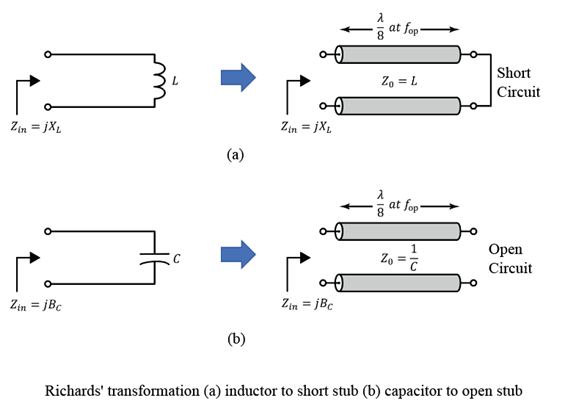# richards

Convert lumped element circuit to distributed element circuit using Richards' transformation

## Syntax

``cktOut = richards(cktIn,opFreq)``
``txOut = richards(LorCobj,opFreq)``
``[txOut,nodes] = richards(LorCobj,opFreq)``
``[txOut,nodes] = richards(___,stubmode=stubType)``

## Description

example

````cktOut = richards(cktIn,opFreq)` applies Richards' transformation on the circuit `cktIn` and returns the circuit object `cktOut` at the given reference frequency `opFreq`. In the `cktOut` all capacitors and inductors are replaced by electrical-length-based transmission line objects `txlineElectricalLength`. NoteYou can apply Richard's transformation only to circuits where all negative terminals of the ports share the same node. ```
````txOut = richards(LorCobj,opFreq)` convert a capacitor or inductor `LorCobj` into an electrical-length-based transmission line object `txOut` at `opFreq`.```

example

````[txOut,nodes] = richards(LorCobj,opFreq)` also returns a vector of suggested nodes `nodes` to connect `txOut` if `LorCobj` is connected to a circuit.```
````[txOut,nodes] = richards(___,stubmode=stubType)` specify the stub type of the output transmission line by using a name-value argument.```

## Examples

collapse all

Create lowpass LC-Pi Chebyshev filter with the passband frequency of 1 GHz, passband attenuation of 0.5 dB, and filter order of 5.

```Fp = 1e9; Ap = 0.5; Ord = 5; cktIn = rffilter("FilterType","Chebyshev","ResponseType","Lowpass","Implementation","LC Pi","PassbandFrequency", ... Fp,"PassbandAttenuation",Ap,"FilterOrder",Ord); opFreq = 1e9;```

Convert the lumped elements of the RF filter to a distributed element using Richards' transformation.

`cktOut = richards(cktIn,opFreq)`
```cktOut = circuit: Circuit element ElementNames: {'C_tx' 'L_tx' 'C_1_tx' 'L_1_tx' 'C_2_tx'} Elements: [1x5 txlineElectricalLength] Nodes: [0 1 2 3 4 5 6] Name: 'unnamed' NumPorts: 2 Terminals: {'p1+' 'p2+' 'p1-' 'p2-'} ```

Create an inductor with the inductance of 5 nH.

`LorCobj= inductor(5e-9);`

Create a circuit.

`ckt = circuit('new_circuit1');`

Add a resistor and the inductor you created earlier to the circuit.

```add(ckt,[1 2],LorCobj); add(ckt,[2 3],resistor(100));```

Set the ports and display the results.

```setports(ckt,[1 0],[3 0]) disp(ckt)```
``` circuit: Circuit element ElementNames: {'L' 'R'} Elements: [1x2 rf.internal.circuit.RLC] Nodes: [0 1 2 3] Name: 'new_circuit1' NumPorts: 2 Terminals: {'p1+' 'p2+' 'p1-' 'p2-'} ```

Apply Richards' transformation to the inductor at 1 GHz and display nodes to connect the transmission line.

`[txOut,nodes] = richards(LorCobj,1e9)`
```txOut = txlineElectricalLength: ElectricalLength element Name: 'L_tx' Z0: 31.4159 LineLength: 0.7854 ReferenceFrequency: 1.0000e+09 Termination: 'Short' StubMode: 'Series' NumPorts: 2 Terminals: {'p1+' 'p2+' 'p1-' 'p2-'} ```
```nodes = 1×4 1 2 0 0 ```

The nodes in this example represent the nodes at which a 2-port transmission line representing the series L. The returned node value is set to `-1` when a ground node cannot be determined from the circuit.

## Input Arguments

collapse all

Inductor or capacitor, specified as an `inductor` or `capacitor` object.

Operating frequency at which the Richards' transformation is applied, specified as a positive scalar.

Stub type of `txOut`, specified as a `'Series'` or `'Shunt'`.

## Output Arguments

collapse all

Output circuit, returned as a `circuit` object.

Electrical-length-based transmission line, returned as a `txlineElectricalLength` object.

Nodes to connect `txOut`, returned as a vector.

## Algorithms

collapse all

### Richards' Transformation

This figure shows how Richards' transformation converts a circuit with capacitors and inductors into an abstract transmission line model .Pozar, David M. Microwave Engineering. 4th ed. Hoboken, NJ: Wiley, 2012.